期刊菜单

Research Progress of Loss Function in Object Detection
DOI: 10.12677/CSA.2021.1111288, PDF, HTML, XML, 下载: 66  浏览: 153  国家自然科学基金支持

Abstract: Loss function is a research hotspot in object detection. With the rapid development of deep learning, object detection algorithms have achieved many research results, and are widely used in face detection, access control recognition, and automatic driving and so forth. In order to further understand the development details of object detection, this article starts from the optimization of the loss function of object detection, combs and summarizes the development process of classification loss and regression loss in object detection in recent years, and classifies and analyzes them. First introduce the development history of classification loss, and then introduce the two major development directions of regression loss: loss function based on Ln norm and based on Intersection over Union (IoU), and analyze the advantages and disadvantages of these loss functions and the correlation between them. Finally, the future development direction of the loss function based on object detection is prospected.

1. 引言

2. 分类损失的研究现状

2.1. Cross Entroy Loss

$p={S}_{i}=\frac{{\text{e}}^{{V}_{i}}}{{\sum }_{i}{\text{e}}^{{V}_{j}}}$

${L}_{CE}=-\mathrm{log}\left({p}_{t}\right)$ (1)

${p}_{t}=\left\{\begin{array}{l}p,\text{\hspace{0.17em}}\text{\hspace{0.17em}}\text{\hspace{0.17em}}\text{\hspace{0.17em}}\text{\hspace{0.17em}}\text{\hspace{0.17em}}\text{\hspace{0.17em}}\text{if}\text{\hspace{0.17em}}y=1\\ 1-p,\text{\hspace{0.17em}}\text{\hspace{0.17em}}\text{otherwise}\end{array}$

2.2. Focal Loss

${L}_{FL}=-{\alpha }_{t}{\left(1-{p}_{t}\right)}^{\gamma }\mathrm{log}\left({p}_{t}\right)$ (2)

${\alpha }_{t}=\left\{\begin{array}{l}\alpha ,\text{\hspace{0.17em}}\text{\hspace{0.17em}}\text{\hspace{0.17em}}\text{\hspace{0.17em}}\text{\hspace{0.17em}}\text{\hspace{0.17em}}\text{\hspace{0.17em}}\text{if}\text{\hspace{0.17em}}y=1\\ 1-\alpha ,\text{\hspace{0.17em}}\text{\hspace{0.17em}}\text{otherwise}\end{array}$

3. 回归损失研究现状

3.1. Ln Loss

Ln Loss是计算候选框与真实框相应参数的Ln范数。真实框的坐标为 $\left({G}_{x},{G}_{y},{G}_{w},{G}_{h}\right)$，候选框的坐标为 $\left({P}_{x},{P}_{y},{P}_{w},{P}_{h}\right)$，网络要学习一个线性映射 $\phi$，使得对候选框进行映射之后的坐标与真实框的坐标无限接近，即 $\phi \left({P}_{x},{P}_{y},{P}_{w},{P}_{h}\right)=\left(\stackrel{^}{{G}_{x}},\stackrel{^}{{G}_{y}},\stackrel{^}{{G}_{w}},\stackrel{^}{{G}_{h}}\right)\approx \left({G}_{x},{G}_{y},{G}_{w},{G}_{h}\right)$。对于线性映射的训练，优化项为：

3.1.1. L1 Loss

L1 Loss又被称为最小绝对误差函数，可以用来度量两个向量之间的差异，损失函数形式如公式(3)所示。

${L}_{1}\left(t\right)=\sum |t|$ (3)

$\frac{\text{d}{L}_{1}\left(t\right)}{\text{d}t}=\left\{\begin{array}{l}1,\text{\hspace{0.17em}}\text{\hspace{0.17em}}\text{\hspace{0.17em}}\text{\hspace{0.17em}}t\ge 0\\ -1,\text{\hspace{0.17em}}\text{\hspace{0.17em}}t<0\end{array}$ (4)

L1 Loss图像如图1蓝色曲线所示，其导数形式如公式(4)所示。由于其梯度是常数，因此在训练过程中可以保持稳定的梯度使得模型得以收敛。也正因为梯度为常数，对于收敛之后的模型，较小的误差也采用同样的梯度，因此在极值点附近容易发生震荡，导致模型难以达到更高的精度。

3.1.2. L2 Loss

L2 Loss又被称为均方误差函数，也是用来度量两个向量之间的差异，与L1 Loss略有不同，损失函数形式如公式(5)所示。

${L}_{2}\left(t\right)=\sum {\left(t\right)}^{2}$ (5)

$\frac{\text{d}{L}_{2}\left(t\right)}{\text{d}t}=2t$ (6)

L2范数函数图像如图1橙色曲线所示，其导数形式如公式(6)所示。函数连续且处处可导，并且随着误差值的减小，梯度也减小，有利于收敛到最小值，但同时训练初期误差值较大，梯度也大，容易产生梯度爆炸导致模型无法收敛，因此使用L2范数作为损失函数时，往往使用较小的学习率从而避免训练初期梯度爆炸。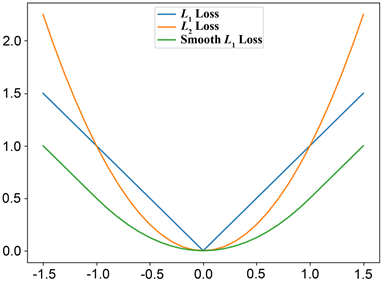Figure 1. Ln Loss function curve

3.1.3. Smooth L1 Loss

Smooth L1 Loss函数形式如公式(7)所示：

$\text{Smooth}{L}_{1}\left(t\right)=\left\{\begin{array}{l}0.5{t}^{2}\text{\hspace{0.17em}}\text{\hspace{0.17em}}\text{\hspace{0.17em}}\text{\hspace{0.17em}}\text{\hspace{0.17em}}\text{\hspace{0.17em}}\text{\hspace{0.17em}}\text{\hspace{0.17em}}\text{\hspace{0.17em}}\text{\hspace{0.17em}}|t|<1\\ |t|-0.5\text{\hspace{0.17em}}\text{\hspace{0.17em}}\text{\hspace{0.17em}}\text{\hspace{0.17em}}\text{\hspace{0.17em}}\text{otherwise}\end{array}$ (7)

$\frac{\text{dSmooth}{L}_{1}\left(t\right)}{\text{d}t}=\left\{\begin{array}{l}t\text{\hspace{0.17em}}\text{\hspace{0.17em}}\text{\hspace{0.17em}}\text{\hspace{0.17em}}\text{\hspace{0.17em}}\text{\hspace{0.17em}}\text{\hspace{0.17em}}\text{\hspace{0.17em}}|t|<1\\ ±1\text{\hspace{0.17em}}\text{\hspace{0.17em}}\text{\hspace{0.17em}}\text{\hspace{0.17em}}\text{otherwise}\end{array}$ (8)

Smooth L1 Loss函数图像如图1绿色曲线所示，其导数形式如公式(8)所示。由求导过程可知，Smooth L1 Loss在损失较大时，按照一个恒定的速率梯度下降，损失较小时，不再按照一个恒定的梯度下降，而是按照变量自身进行动态调整。Smooth L1 Loss结合了L1 Loss与L2 Loss的优点：模型开始训练时，损失较大，采用L1 Loss的形式，使网络保持稳健的梯度；模型收敛时，当损失较小时采用L2 Loss的形式，使模型可以收敛到更高的精度。

3.1.4. Balanced L1 Loss

$\frac{\text{d}{L}_{b}\left(t\right)}{\text{d}t}=\left\{\begin{array}{l}\alpha \mathrm{ln}\left(b|t|+1\right)\text{\hspace{0.17em}}\text{\hspace{0.17em}}\text{\hspace{0.17em}}\text{\hspace{0.17em}}\text{\hspace{0.17em}}\text{\hspace{0.17em}}\text{\hspace{0.17em}}|t|<1\\ \gamma \text{\hspace{0.17em}}\text{\hspace{0.17em}}\text{\hspace{0.17em}}\text{\hspace{0.17em}}\text{\hspace{0.17em}}\text{\hspace{0.17em}}\text{\hspace{0.17em}}\text{\hspace{0.17em}}\text{\hspace{0.17em}}\text{\hspace{0.17em}}\text{\hspace{0.17em}}\text{\hspace{0.17em}}\text{\hspace{0.17em}}\text{\hspace{0.17em}}\text{\hspace{0.17em}}\text{\hspace{0.17em}}\text{\hspace{0.17em}}\text{\hspace{0.17em}}\text{\hspace{0.17em}}\text{otherwise}\end{array}$ (9)

${L}_{b}\left(t\right)=\left\{\begin{array}{l}\frac{\alpha }{b}\left(b|t|+1\right)\mathrm{ln}\left(b|t|+1\right)-\alpha |t|\text{\hspace{0.17em}}\text{\hspace{0.17em}}\text{\hspace{0.17em}}\text{\hspace{0.17em}}\text{if}\text{\hspace{0.17em}}|t|<1\\ \gamma |t|+C\text{\hspace{0.17em}}\text{\hspace{0.17em}}\text{\hspace{0.17em}}\text{\hspace{0.17em}}\text{\hspace{0.17em}}\text{\hspace{0.17em}}\text{\hspace{0.17em}}\text{\hspace{0.17em}}\text{\hspace{0.17em}}\text{\hspace{0.17em}}\text{\hspace{0.17em}}\text{\hspace{0.17em}}\text{\hspace{0.17em}}\text{\hspace{0.17em}}\text{\hspace{0.17em}}\text{\hspace{0.17em}}\text{\hspace{0.17em}}\text{\hspace{0.17em}}\text{\hspace{0.17em}}\text{\hspace{0.17em}}\text{\hspace{0.17em}}\text{\hspace{0.17em}}\text{\hspace{0.17em}}\text{\hspace{0.17em}}\text{\hspace{0.17em}}\text{\hspace{0.17em}}\text{\hspace{0.17em}}\text{\hspace{0.17em}}\text{\hspace{0.17em}}\text{otherwise}\end{array}$ (10)

3.2. IoU-Based Loss

3.2.1. IoU Loss

$\text{IoU}\left(P,G\right)=\frac{P\cap G}{P\cup G}$ (11)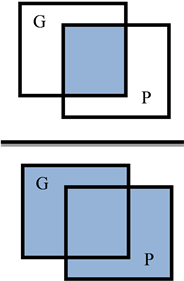Figure 2. IoU schematic diagram

IoU Loss的损失函数如公式(12)所示，易知IoU的取值范围是[0, 1]，IoU的值越接近1，说明候选框与真实框越接近，定位越准确，此时损失函数值越小，惩罚也就越小，反之惩罚越大。但同时也可看出IoU Loss的缺点是当候选框与真实框不相交时，IoU为0，LIoU为常数1，梯度无法回传。

${L}_{\text{IoU}}=1-\text{IoU}\left(P,G\right)$ (12)

3.2.2. GIoU Loss

${L}_{\text{GIoU}}=1-\text{IoU}+\frac{|C-P\cup G|}{|C|}$ (13)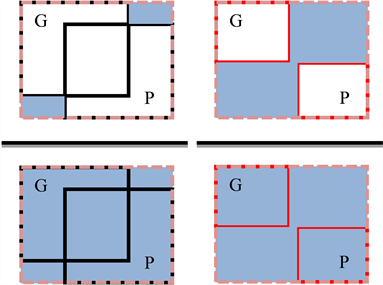Figure 3. GIoU schematic diagram of penalty items

3.2.3. DIoU Loss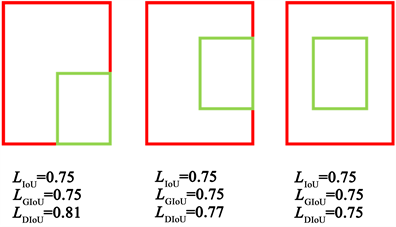Figure 4. The instances of GIoU unable to regression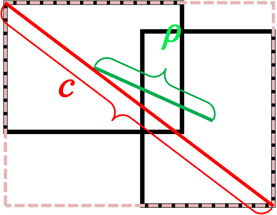Figure 5. DIoU schematic diagram of penalty items

${L}_{\text{DIoU}}=1-\text{IoU}+\frac{{\rho }^{2}}{{c}^{2}}$ (14)

3.2.4. CIoU Loss

CIoU Loss认为候选框与真实框进行匹配的几何要素包括面积、中心点距离、长宽比例，这三部分决定了候选框的定位精确度。DIoU Loss已经包含了面积与中心点距离，因此CIoU Loss将两框的长宽比也作为惩罚项加入损失函数中，如公式(15)所示：

${L}_{\text{CIoU}}=1-\text{IoU}+\frac{{\rho }^{2}}{{c}^{2}}+\alpha v$ (15)

3.2.5. Focal EIoU Loss

${L}_{\text{EIoU}}=1-\text{IoU}+\frac{{\rho }^{2}}{{c}^{2}}+\frac{{\rho }^{2}\left(w,{w}^{gt}\right)}{{C}_{w}^{2}}+\frac{{\rho }^{2}\left(h,{h}^{gt}\right)}{{C}_{h}^{2}}$ (16)

${L}_{\text{FocalEIoU}}={\text{IoU}}^{\gamma }{L}_{\text{EIoU}}$ (17)

4. 总结与展望

  Girshick, R., Donahue, J., Darrell, T., et al. (2014) Rich Feature Hierarchies for Accurate Object Detection and Semantic Segmentation. IEEE Conference on Computer Vision and Pattern Recognition, Columbus, 23-28 June 2014, 580-587. https://doi.org/10.1109/CVPR.2014.81  Girshick, R. (2015) Fast R-CNN. IEEE International Conference on Computer Vision (ICCV), Santiago, 7-13 December 2015, 1440-1448. https://doi.org/10.1109/ICCV.2015.169  Ren, S., He, K., Girshick, R., et al. (2015) Faster R-CNN: Towards Real-Time Object Detection with Region Pro-posal Networks. IEEE Transactions on Pattern Analysis and Machine Intelligence, 39, 1137-1149. https://doi.org/10.1109/TPAMI.2016.2577031  Cai, Z.W. and Vasconcelos, N. (2017) Cascade R-CNN: Delving into High Quality Object Detection. 2018 IEEE/CVF Conference on Computer Vision and Pattern Recognition, Salt Lake City, 18-23 June 2018, 6154-6162. https://doi.org/10.1109/CVPR.2018.00644  He, K.M., Georgia, G., Piotr, D., et al. (2018) Mask R-CNN. IEEE Transactions on Pattern Analysis & Machine Intelligence, 42, 386-397. https://doi.org/10.1109/TPAMI.2018.2844175  Pang, J.M., Chen, K., Shi, J.P., et al. (2019) Libra R-CNN: Towards Balanced Learning for Object Detection. 2019 IEEE/CVF Conference on Computer Vision and Pattern Recog-nition (CVPR), Long Beach, 15-20 June 2019, 821-830. https://doi.org/10.1109/CVPR.2019.00091  Sun, P., Zhang, R.F., Jiang, Y., et al. (2021) Sparse R-CNN: End-to-End Object Detection with Learnable Proposals. 2021 IEEE/CVF Conference on Computer Vision and Pattern Recognition (CVPR), Nashville, 20-25 June 2021, 14449-14458. https://doi.org/10.1109/CVPR46437.2021.01422  Redmon, J., Divvala, S., Girshick, R., et al. (2016) You Only Look Once: Unified, Real-Time Object Detection. 2016 IEEE Conference on Computer Vision and Pattern Recognition (CVPR), Las Vegas, 27-30 June 2016, 779-788. https://doi.org/10.1109/CVPR.2016.91  Redmon, J. and Farhadi, A. (2016) YOLO9000: Better, Faster, Stronger. 2016 IEEE Conference on Computer Vision and Pattern Recognition (CVPR), Las Vegas, 27-30 June 2016, 6517-6525. https://doi.org/10.1109/CVPR.2017.690  Redmon, J. and Farhadi, A. (2018) YOLOv3: An In-cremental Improvement. https://arxiv.org/abs/1804.02767  Bochkovskiy, A., et al. (2020) YOLOv4: Optimal Speed and Accuracy of Object Detection. https://arxiv.org/abs/2004.10934  Liu, W., Anguelov, D., Erhan, D., et al. (2016) SSD: Single Shot Multi-Box Detector. 2016 IEEE Conference on Computer Vision and Pattern Recognition (CVPR), Las Vegas, 27-30 June 2016, 21-37. https://doi.org/10.1007/978-3-319-46448-0_2  Fu, C.Y., Liu, W., Ranga, A., et al. (2017) DSSD: Deconvolu-tional Single Shot Detector. https://arxiv.org/abs/1701.06659  Carion, N., Massa, F., Synnaeve, G., et al. End-to-End Object Detection with Transformers. https://arxiv.org/abs/2005.12872  鲁晨光. Shannon公式改造[J]. 通信学报, 1991, 12(2): 95-96.  Lin, T.Y., Goyal, P., Girshick, R., He, K.M., et al. (2018) Focal Loss for Dense Object Detection. 2017 IEEE International Conference on Computer Vision (ICCV), Venice, 22-29 October 2017, 2999-3007. https://doi.org/10.1109/ICCV.2017.324  Yu, J.H., Jiang, Y., Wang, Z.Y., et al. (2016) Unitbox: An Advanced Object Detection Network. Proceedings of the 24th ACM International Conference on Multimedia, Amsterdam, 15-19 October 2016, 516-520. https://doi.org/10.1145/2964284.2967274  Rezatofighi, H., Tsoi, N., Gwak, J.Y., et al. (2019) Generalized Intersection over Union: A Metric and a Loss for Bounding Box Regression. IEEE Conference on Computer Vision and Pattern Recognition (CVPR), Long Beach, 15-20 June 2019, 658-666. https://doi.org/10.1109/CVPR.2019.00075  Zheng, Z., Wang, P., Liu, W., et al. (2020) Distance-IOU Loss: Faster and Better Learning for Bounding Box Re-gression. The Association for the Advance of Artificial Intelligence (AAAI), New York, 7-12 February 2020, 12993-13000.  Zhang, Y.F., Ren, W.Q., Zhang, Z., et al. (2021) Focal and Efficient IOU Loss for Accurate Bounding Box Regression. https://arxiv.org/abs/2101.08158